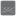• # factorial (length)

## Question related to missionReversed Permutation Index

My code can pass the check if I wrote as below, but not work if wrote in CODEB as below when length is GE 24, what's the problem inside?

CODEB : diff = (((math.factorial(length))*interval)) /length

```\`    FULL CODE:\`
\`from typing import Iterable\`
\`import math\`
\`\`
\`def reversed_permutation_index(length: int, index: int):\`
\`    num_list=list(range(length))\`
\`    result=[]\`
\`    while (length>0 ):\`
\`        interval = math.ceil((length*index/math.factorial(length))) -1\`
\`        diff = (((math.factorial(length-1))*interval))\`
\`        index -=diff\`
\`        print (interval, diff)\`
\`        result.append(num_list[interval])\`
\`        num_list.remove(num_list[interval])\`
\`        length-=1\`
\`    return result\`
```16